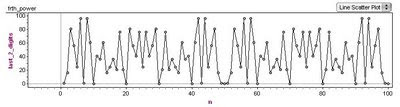## Thursday, July 17, 2008

### Digit Patterns in Square Numbers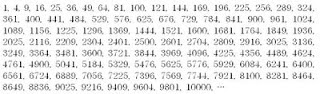If you take a look at the square numbers (n^2, n a positive integer), you'll notice plenty of patterns in the digits. For example, if you look at just the last digit of each square, you'll observe the repeating pattern 1, 4, 9, 6, 5, 6, 9, 4, 1, 0, ... If you construct a graph of "last digit" vs n (like the one below, built with Fathom), the symmetry and period of this digit pattern is apparent.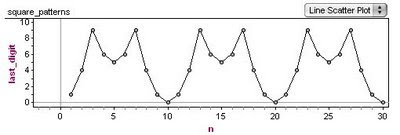Why does this happen? The periodic nature of the pattern is easy to understand - when you square a number, only the digit in the ones place contributes to ones place of the product. For example, 22*22 and 32*32 are both going to have a 4 as their last digit - the values in the tens place (or any other place other than the ones) do not affect what ends up as the last digit.

The reason for the symmetry about n=5 is a little less obvious. To see what is going on, it is helpful to use modular arithmetic and to realize that " last digit of n" is the same as "n mod 10". Considering what 10-n looks like mod 10 after it is squared, we have the equation below.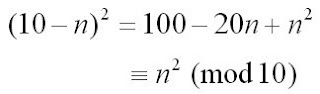This tells us that the last digit of (10-n)^2 is the same as the last digit of n^2, because everything else that is different about these two numbers is divisible by 10.

If you look at the last two digits of the square numbers, you see another repeating pattern that has similar symmetries.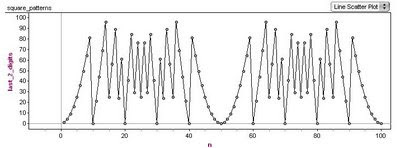This is a nice looking graph - the period is 50 with a line of symmetry at n=25. You can think about it in the same way as the one-digit case, this time the symmetry is understood by looking at (50-n)^2 mod 100. (Looking at numbers mod 100 tells us their last two digits.)

If you decide to investigate patterns in cubes or higher powers, you'll see somewhat similar results. Using the binomial theorem and modular arithmetic, you can see why even powers give symmetry similar to the n^2 case, while odd powers do not (although all are periodic).

This graph shows the pattern in the last digit of n^3.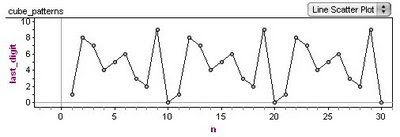This last graph shows the pattern for the last two digits of n^4.